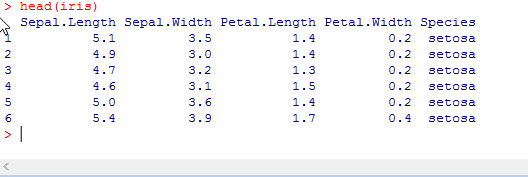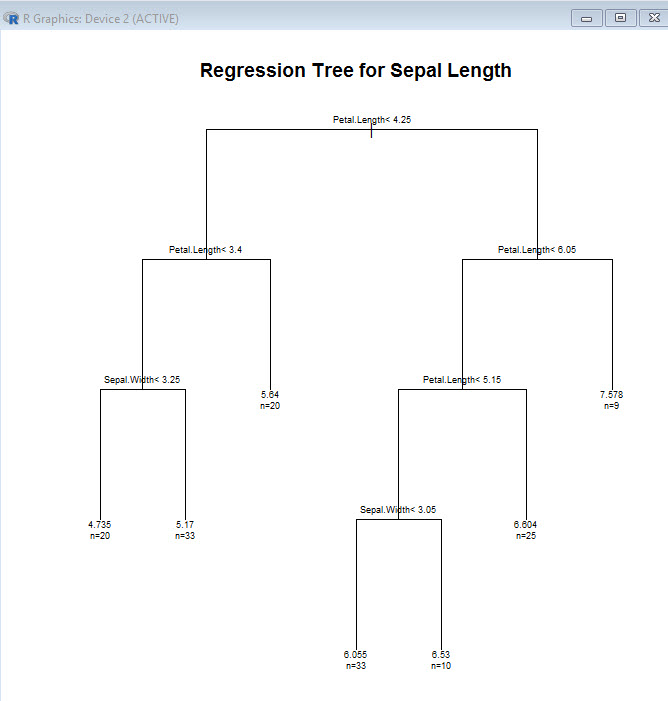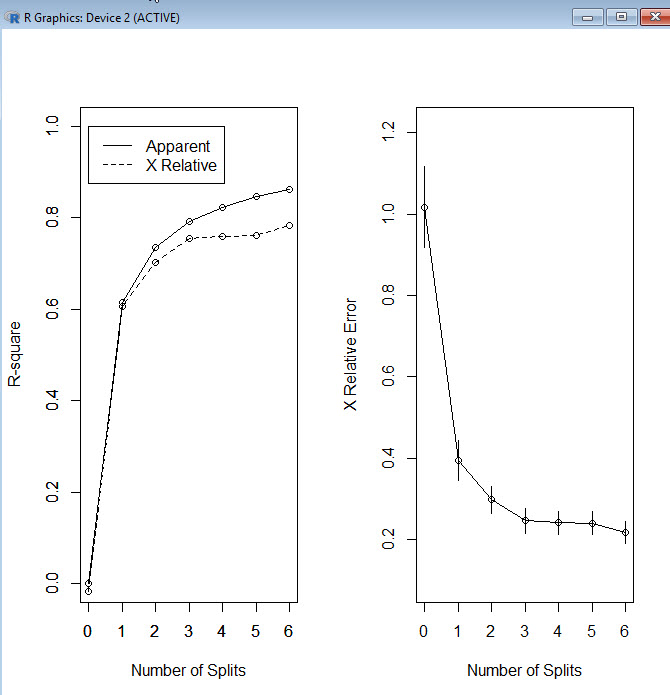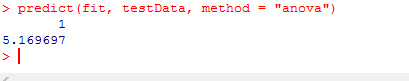# R: Decision Trees (Regression)

Decision Trees are popular supervised machine learning algorithms. You will often find the abbreviation CART when reading up on decision trees. CART stands for Classification and Regression Trees.

In this example we are going to create a Regression Tree. Meaning we are going to attempt to build a model that can predict a numeric value.

We are going to start by taking a look at the data. In this example we are going to be using the Iris data set native to R. This data set

`iris`As you can see, our data has 5 variables – Sepal.Length, Sepal.Width, Petal.Length, Petal.Width, and Species. The first 4 variables refer to measurements of flower parts and the species identifies which species of iris this flower represents.

In the Classification example, we tried to predict the Species of flower. In this example we are going to try to predict the Sepal.Length

In order to build our decision tree, first we need to install the correct package.

```install.packages("rpart")

library(rpart)```

Next we are going to create our tree. Since we want to predict Sepal.Length – that will be the first element in our fit equation.

```fit <- rpart(Sepal.Length ~ Sepal.Width + Petal.Length + Petal.Width+ Species,
method="anova", data=iris )```

Note the method in this model is anova. This means we are going to try to predict a number value. If we were doing a classifier model, the method would be class.

Now let’s plot out our model

```plot(fit, uniform=TRUE,
main="Regression Tree for Sepal Length")
text(fit, use.n=TRUE, cex = .6)```

Note the splits are marked – like the top split is Petal.Length < 4.25

Also, at the terminating point of each branch, you see and n= . The number following this is the number of elements from the data file that fit at the end of that branch.While this model actually works out pretty good, one thing to look for is over fitting. A good sign of that would be having a bunch of branches terminating with n values of 1 or 2. This means the model is tuned too much to the test data and when run up against a new set of data it will most likely result in poor predictions.

Of course we can look at some of the numbers if you are so inclined.Notice the xerror (cross validation error) gets better with each split. That is something you want to look out for. If that number starts to creep up as the splits increase, that is a sign you may want to prune some of the branches. I will show how to do that in another lesson.

To get a better picture of the change in xerror as the splits increase, let’s look at a new visualization

```par(mfrow=c(1,2))
rsq.rpart(fit)```

This produces 2 charts, 1rst on shows how R-Squared improves as splits increase (remember R-squared gets better as it approaches 1 so this model is improving with each spit)

The second chart shows how xerror decreases with each split. For models that need pruning, you would see the curve starting to go back up as the splits increase. Imagine is split 6 was higher than split 5.Okay, so finally now that we know the model is good, let’s make a prediction.

```testData  <-data.frame (Species = 'setosa', Sepal.Width = 4, Petal.Length =1.2,
Petal.Width=0.3)
predict(fit, testData, method = "anova")```So as you can see, based on our test data, the model predicts our Sepal.Length will be approx 5.17.